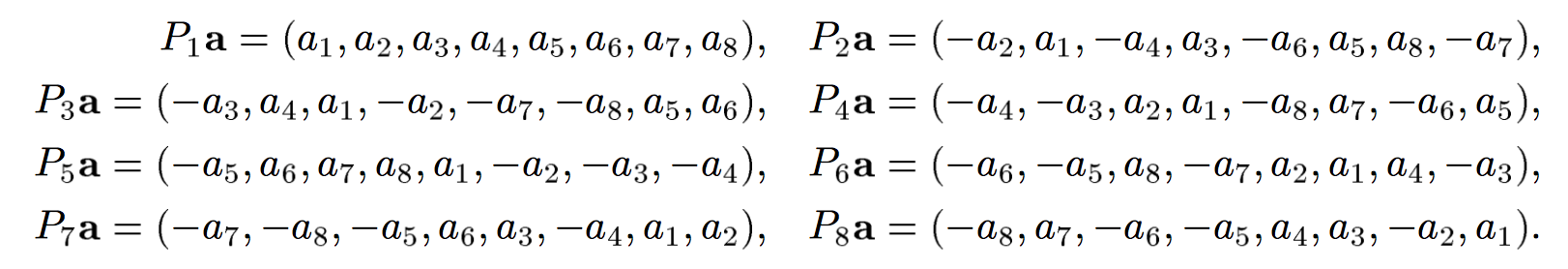maximum of orthogonal vectors

$$v_1=(x_1,x_2,x_3\cdot\cdot\cdot,x_n)$$is such a vector. By changing its signs and positions of each component $x_i$, we can get different vectors. When n is odd, it's impossible for any of orthogonal to each other. When n is even, what is the maximum number of vectors that are orthogonal to each other.

For example, vectors $(x_1, x_2, x_3, x_4)$, $(-x_2,x_1,x_4,-x_3)$ and $(x_3,x_4,-x_1,-x_2)$ are mutually perpendicular when $n$=$4$.

What about $n=4,6,8,10,...$ and how many?

This construction was studied in On systems of orthogonal permutations, where it was proven that a complete set of $n$ mutually orthogonal vectors of length $n$ exists only for $n=1,2,4,8$. For $n=4$ the four permutations are $(x_1,x_2,x_3,x_4)$, $(-x_2,x_1,-x_4,x_3)$, $(-x_3,x_4,x_1,-x_2)$, and $(-x_4,-x_3,x_2,x_1)$; for $n=8$ the eight permutations are given in the cited paper asTo answer the OP, my conclusion from this construction is that the maximum number $m$ of orthogonal permutations of a length $n$ vector cannot exceed 8, and for $n=2,4,6,8\ldots$ cycles through $m=2,4,2,8,2,4,2,8,\ldots$.
• @Carlo Beenakker so not all of the $2^n$, just 1,2,4,8? – Mclalalala Mar 10 '17 at 13:49
• But I suppose that all of $2^n$ is available – Mclalalala Mar 10 '17 at 13:50